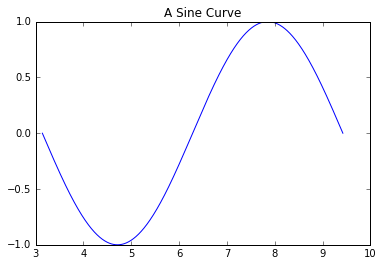Show Sidebar Hide Sidebar# Modifying Matplotlib Figure in matplotlib

How to modify a matplotlib figure to a Plotly Figure

Note: this page is part of the documentation for version 3 of Plotly.py, which is not the most recent version.
See our Version 4 Migration Guide for information about how to upgrade.

# Modifying a Matplotlib Figure¶

In this example, we will see how we can convert a Matplotlib's Plot to a Plotly Compatible Figure object.

Importing the dependencies:

In :
%matplotlib inline
import pprint
import numpy as np

# Matplotlib
import matplotlib.pyplot as plt

# Plotly
import plotly.plotly as py
import plotly.tools as tls


We will create a simple sine curve using Matplotlib. Then, we will convert it into Plotly's Figure object, and add another curve to the plot. Finally we will be able to plot the curve using Plotly.

In :
## Generating the data..
x =  np.linspace(np.pi, 3*np.pi, 1000)
sinx = np.sin(x)
logx = np.log(x)

In :
# Creating the matplotlib graph..
mpl_fig = plt.figure()
ax = mpl_fig.add_subplot(111)
ax.plot(x, sinx)
ax.set_title('A Sine Curve')

Out:
<matplotlib.text.Text at 0x10baafd90>Now we will convert the figure into Plotly compatible figure object using a utility method mpl_to_plotly.

In :
help (tls.mpl_to_plotly)

Help on function mpl_to_plotly in module plotly.tools:

mpl_to_plotly(fig, resize=False, strip_style=False, verbose=False)
Convert a matplotlib figure to plotly dictionary and send.

All available information about matplotlib visualizations are stored
within a matplotlib.figure.Figure object. You can create a plot in python
using matplotlib, store the figure object, and then pass this object to
the fig_to_plotly function. In the background, mplexporter is used to
crawl through the mpl figure object for appropriate information. This
information is then systematically sent to the PlotlyRenderer which
creates the JSON structure used to make plotly visualizations. Finally,
these dictionaries are sent to plotly and your browser should open up a
new tab for viewing! Optionally, if you're working in IPython, you can
set notebook=True and the PlotlyRenderer will call plotly.iplot instead
of plotly.plot to have the graph appear directly in the IPython notebook.

Note, this function gives the user access to a simple, one-line way to
render an mpl figure in plotly. If you need to trouble shoot, you can do
this step manually by NOT running this fuction and entereing the following:

===========================================================================
from mplexporter import Exporter
from mplexporter.renderers import PlotlyRenderer

# create an mpl figure and store it under a varialble 'fig'

renderer = PlotlyRenderer()
exporter = Exporter(renderer)
exporter.run(fig)
===========================================================================

You can then inspect the JSON structures by accessing these:

renderer.layout -- a plotly layout dictionary
renderer.data -- a list of plotly data dictionaries

Positional arguments:
fig -- a matplotlib figure object
username -- a valid plotly username **
api_key -- a valid api_key for the above username **
notebook -- an option for use with an IPython notebook

** Don't have a username/api_key? Try looking here:
https://plot.ly/plot

** Forgot your api_key? Try signing in and looking here:
https://plot.ly/python/getting-started


In :
# Converting to Plotly's Figure object..
plotly_fig = tls.mpl_to_plotly(mpl_fig)

In :
pp = pprint.PrettyPrinter(indent=4)
pp.pprint(plotly_fig['layout'])

{   'autosize': False,
'height': 320,
'hovermode': 'closest',
'margin': {   'b': 40, 'l': 60, 'pad': 0, 'r': 47, 't': 31},
'showlegend': False,
'title': u'A Sine Curve',
'titlefont': {   'color': '#000000', 'size': 12.0},
'width': 480,
'xaxis1': {   'anchor': 'y1',
'domain': [0.0, 1.0],
'mirror': 'ticks',
'nticks': 8,
'range': [3.0, 10.0],
'showgrid': False,
'showline': True,
'side': 'bottom',
'tickfont': {   'size': 10.0},
'ticks': 'inside',
'type': u'linear',
'zeroline': False},
'yaxis1': {   'anchor': 'x1',
'domain': [0.0, 1.0],
'mirror': 'ticks',
'nticks': 5,
'range': [-1.0, 1.0],
'showgrid': False,
'showline': True,
'side': 'left',
'tickfont': {   'size': 10.0},
'ticks': 'inside',
'type': u'linear',
'zeroline': False}}


Now, we will supply some data to plotly_fig:

In :
# Adding some new data points ..
plotly_fig['data'].append( dict(x=x, y=logx, type='scatter', mode='lines') )


Similarly, we can also edit the Layout. We will edit the Plot Title, and enable the grid here:

In :
plotly_fig['layout']['title'] = 'Sine and Logarithmic Curve'

plotly_fig['layout']['xaxis1']['showgrid'] = True
plotly_fig['layout']['xaxis1']['autorange'] = True

plotly_fig['layout']['yaxis1']['showgrid'] = True
plotly_fig['layout']['yaxis1']['autorange'] = True


Now we can plot the modified figure using Plotly's Graphing API:

In :
py.iplot(plotly_fig, filename='sine-and-logarithmic-plot')

Out: